## interrupted time series

`hi!I have  a data ( number of  crashes ). I used interrupted time series to show the effect of speed camera that introduced at time=49I want to plot in one graph as in the Figure1a in the link http://www.cdc.gov/pcd/issues/2013/12_0268.htm 30 1 26 239 3 44 4 35 5     20 6 27 7 67 8 25 9 39 10 69 11   74 12 45 13 41 14 50 15 67 16 55 17   77 18 41 19 38 2038 21 46 22 45 23   62 24  60 25  62 26 49 27 60 2878 29         69 30      64 31 86 3268 33 53 34 59 35 56 36 68 37 57 38 59 39 101 40 63 4190 42 92 43 64 44      77 45 69 4682 47 70 48 63 49 66 50  78 51 94 52 88 53 62 54 77 55 92 56 76 57 55 58 70 59 54 60 76 61 58 62 58 6379 64 77 65 79 66 ;`
shayma
1 ACCEPTED SOLUTION

Accepted Solutions

## Re: interrupted time series

Here's one "brute force" way to do it (somebody might come up with a more elegant way to do it). It produces the following plot: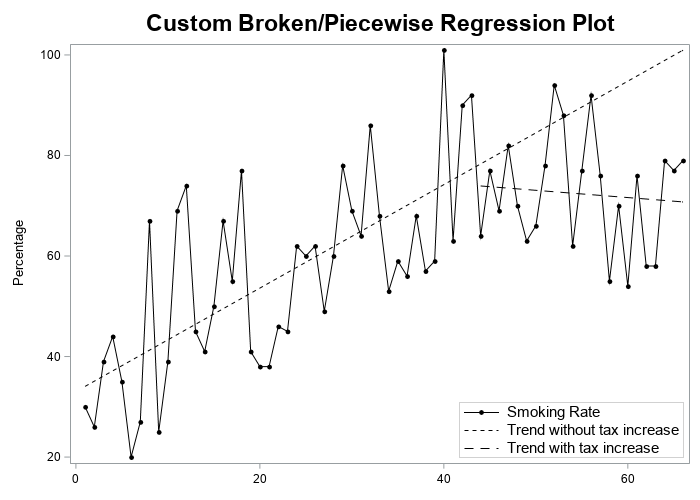data my_data;
format time comma8.0 value comma8.0;
input value time;
if time <=43 then series1=value;
else series2=value;
datalines;
30 1
26 2
39 3
44 4
35 5
20 6
27 7
67 8
25 9
39 10
69 11
74 12
45 13
41 14
50 15
67 16
55 17
77 18
41 19
38 20
38 21
46 22
45 23
62 24
60 25
62 26
49 27
60 28
78 29
69 30
64 31
86 32
68 33
53 34
59 35
56 36
68 37
57 38
59 39

101 40
63 41
90 42
92 43
64 44
77 45
69 46
82 47
70 48
63 49
66 50
78 51
94 52
88 53
62 54
77 55
92 56
76 57
55 58
70 59
54 60
76 61
58 62
58 63
79 64
77 65
79 66
;
run;

proc reg data=my_data plots=none noprint;
model series1 = time;
output out=my_data p=series1_predicted;
run;

proc reg data=my_data plots=none noprint;
model series2 = time;
output out=my_data p=series2_predicted;
run;

data my_data; set my_data;
if time<=43 then series2_predicted=.;
run;

title1 height=16pt "Custom Broken/Piecewise Regression Plot";

proc sgplot data=my_data;
label value='Smoking Rate';
label series1_predicted='Trend without tax increase';
label series2_predicted='Trend with tax increase';
series x=time y=value / lineattrs=(color=black)
markers markerattrs=(size=6px color=black symbol=circlefilled);
series x=time y=series1_predicted / lineattrs=(color=black pattern=shortdash);
series x=time y=series2_predicted / lineattrs=(color=black pattern=dash);
yaxis label="Percentage";
xaxis display=(nolabel);
keylegend / across=1 position=bottomright location=inside
linelength=35px border valueattrs=(size=11pt);
run;

13 REPLIES 13

## Re: interrupted time series

I am not sure what question you are asking. You can use PROC SGPLOT to create the plot of NumCrashes vs Time:

``````proc sgplot data=Have;
series y=NumCrashes x=Time / markers;
run;
``````## Re: interrupted time series

thank you

I used intervention analysis. so, i need to plot a graph similar to the  picture below.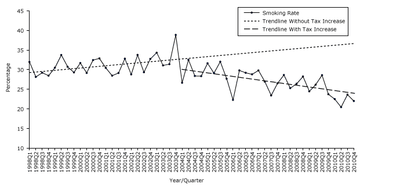shayma

## Re: interrupted time series

OK, so add the two fit lines to the data set that has the data. Use a binary indicator variable to specify which predicted values are for the pre/post model. Then add another SERIES statement, like this

series x=Time y=Predicted / group=PrePost;

For example:

``````proc sort data=Sashelp.Class out=Have;
by Height;
run;

data Pred;
input PrePost Height Predicted;
datalines;
1 51  56
1 72 150
2 64 110
2 72 100
;

data All;
set Have Pred;
run;

proc sgplot data=All;
series x=Height y=Weight;
series x=Height y=Predicted / group=PrePost;
run;``````

## Re: interrupted time series

ok, I need the regression lines in the graph!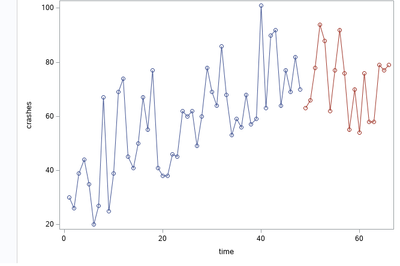shayma

## Re: interrupted time series

Do you have the regression lines or not? In your original post you said "I used interrupted time series to show the effect of ...," which sounds like you already have the regression line.

If you want OLS lines for each segment, you can use

REG x=Time y=NumCrashes / group=PrePost;

Be aware, however, that those are least squares regression lines. If you want something more sophisticated, fit a model to the data and use the OUTPUT statement to output the predicted values to a SAS data set, then use my earlier method.

## Re: interrupted time series

i used this code

`proc sgplot data=FitOut;scatter x=time y=crashes / group=group;series x=time y=crashes/ group=group;reg x=time y=crashes/ group=group;run; `

and the result as show below. I used linear regression and I have the equation of the regression but I want to show the regression lines in the graph in two period ( before and after )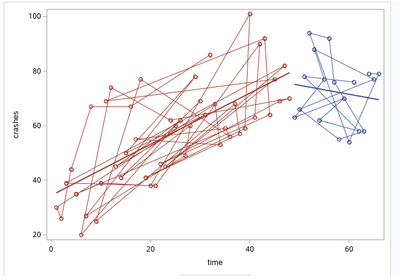shayma

## Re: interrupted time series

Notice the PROC SORT at the top of my example. Try this:

``````proc sort data=FitOut;
by group time;
run;

proc sgplot data=FitOut;
series x=time y=crashes/ group=group markers;
reg x=time y=crashes/ group=group nomarkers;
run;``````

## Re: interrupted time series

Thank you, this what i asked for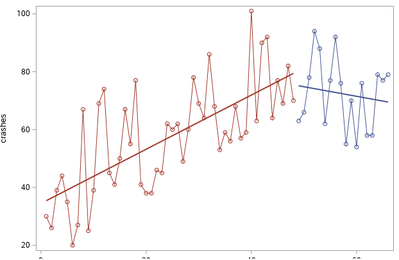if possible, I want to delete the regression line for the first period and make it for all period (1-66) .how?

shayma

## Re: interrupted time series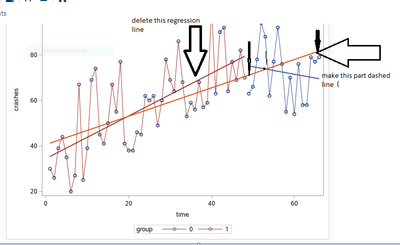shayma

## Re: interrupted time series

Here's one "brute force" way to do it (somebody might come up with a more elegant way to do it). It produces the following plot:data my_data;
format time comma8.0 value comma8.0;
input value time;
if time <=43 then series1=value;
else series2=value;
datalines;
30 1
26 2
39 3
44 4
35 5
20 6
27 7
67 8
25 9
39 10
69 11
74 12
45 13
41 14
50 15
67 16
55 17
77 18
41 19
38 20
38 21
46 22
45 23
62 24
60 25
62 26
49 27
60 28
78 29
69 30
64 31
86 32
68 33
53 34
59 35
56 36
68 37
57 38
59 39

101 40
63 41
90 42
92 43
64 44
77 45
69 46
82 47
70 48
63 49
66 50
78 51
94 52
88 53
62 54
77 55
92 56
76 57
55 58
70 59
54 60
76 61
58 62
58 63
79 64
77 65
79 66
;
run;

proc reg data=my_data plots=none noprint;
model series1 = time;
output out=my_data p=series1_predicted;
run;

proc reg data=my_data plots=none noprint;
model series2 = time;
output out=my_data p=series2_predicted;
run;

data my_data; set my_data;
if time<=43 then series2_predicted=.;
run;

title1 height=16pt "Custom Broken/Piecewise Regression Plot";

proc sgplot data=my_data;
label value='Smoking Rate';
label series1_predicted='Trend without tax increase';
label series2_predicted='Trend with tax increase';
series x=time y=value / lineattrs=(color=black)
markers markerattrs=(size=6px color=black symbol=circlefilled);
series x=time y=series1_predicted / lineattrs=(color=black pattern=shortdash);
series x=time y=series2_predicted / lineattrs=(color=black pattern=dash);
yaxis label="Percentage";
xaxis display=(nolabel);
keylegend / across=1 position=bottomright location=inside
linelength=35px border valueattrs=(size=11pt);
run;

## Re: interrupted time series

@GraphGuy  thank you, you are right, this is a Piecewise Regression

but how can get the coefficients (regression equation ) for the Piecewise Regression and R square  by sas ?

shayma

## Re: interrupted time series

All of your questions can be answered by a Google search or by reading the SAS documentation (especially the Getting Started examples) for PROC GLM. There are many blog posts about regression models that change at a cutpoint.

There are also papers about piecewise linear models.Keywords are

+SAS piecewise linear regression model